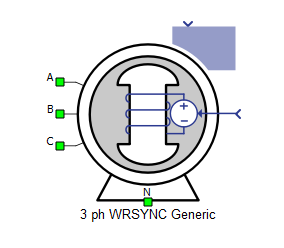# Three Phase Wound Rotor Synchronous Machine (Generic)

Description of the Three Phase Wound Rotor Synchronous Machine (Generic) component in Schematic Editor.

This simplified model of a three phase wound rotor synchronous machine allows simulation of machine dynamics in real time using only generic machine data such as power and voltage. It is built as an easy to use and implement, signal-processing-based component.

Table 1. Three Phase Wound Rotor Synchronous Machine (Generic) component in Typhoon HIL Schematic Editor
component component dialog window component parameters• Property tabs:

A, B, and C are the stator winding terminals. The stator winding uses the voltage behind reactance interface. The stator winding arrangement (connection) is considered to be star connected with an accessible neutral point. The inputs to the system are the field winding voltage and mechanical source (which can be selected to be either mechanical torque or speed). Both inputs to the model are in the form of signals.

In the simplified model of the machine, the rotor is assumed to be round and the field winding is assumed to be decoupled and operating independently. Meanwhile, the machine has only one damper winding in the q-axis which is short-circuited without accessible terminals.

## Electrical sub-system model

The electrical part of the machine is represented by the following system of equations, modeled in the rotating dq reference frame. The dq reference frame is attached to the rotor, and the direct axis is aligned with the field winding axis. The modeled dynamics can be represented with the following equations:

$\left[\begin{array}{c}{v}_{ds}\\ {v}_{qs}\\ {v}_{kq}\\ {v}_{fd}\end{array}\right]=\left[\begin{array}{cccc}{R}_{s}& 0& 0& 0\\ 0& {R}_{s}& 0& 0\\ 0& 0& {R}_{kq}& 0\\ 0& 0& 0& {R}_{fd}\end{array}\right]\left[\begin{array}{c}{i}_{ds}\\ {i}_{qs}\\ {i}_{kq}\\ {i}_{fd}\end{array}\right]+\frac{d}{dt}\left[\begin{array}{c}{\psi }_{ds}\\ {\psi }_{qs}\\ {\psi }_{kq}\\ {\psi }_{fd}\end{array}\right]+\left[\begin{array}{c}{-\omega }_{r}{\psi }_{qs}\\ {\omega }_{r}{\psi }_{ds}\\ 0\\ 0\end{array}\right]$

$\left[\begin{array}{c}{\psi }_{ds}\\ {\psi }_{qs}\\ {\psi }_{kq}\\ {\psi }_{fd}\end{array}\right]=\left[\begin{array}{cccc}{L}_{ls}+{L}_{md}& 0& 0& {L}_{md}\\ 0& {L}_{ls}+{L}_{mq}& {L}_{mq}& 0\\ 0& {L}_{mq}& {L}_{lkq}+{L}_{mq}& 0\\ 0& 0& 0& {L}_{lfd}+{L}_{md}\end{array}\right]\left[\begin{array}{c}{i}_{ds}\\ {i}_{qs}\\ {i}_{kq}\\ {i}_{fd}\end{array}\right]$

${T}_{e}=\frac{3}{2}p\left({\psi }_{ds}{i}_{qs}-{\psi }_{qs}{i}_{ds}\right)$

In this generic model of the synchronous machine, the machine electrical parameters are approximated based on the machine power, voltage, frequency, and speed to be as close to realistic values as possible.

Table 2. Electrical sub-system model variables
Symbol Description
ψds Direct axis component of the stator flux [Wb]
ψqs Quadrature axis component of the stator flux [Wb]
ψkd Direct axis component of the damper winding flux, referred to the stator [Wb]
ψkq Quadrature axis component of the damper winding flux, referred to the stator [Wb]
ψfd Field winding flux, referred to the stator [Wb]
ids Direct axis component of the stator current [A]
iqs Quadrature axis component of the stator current [A]
ikd Direct axis component of the damper winding current, referred to the stator [A]
ikq Quadrature axis component of the damper winding current, referred to the stator [A]
ifd Field winding current, referred to the stator [A]
vds Direct axis component of the stator voltage [V]
vqs Quadrature axis component of the stator voltage [V]
vkd Direct axis component of the damper winding voltage, referred to the stator [V]
vkq Quadrature axis component of the damper winding voltage, referred to the stator [V]
vfd Field winding voltage, referred to the stator [V]
Rs Stator phase resistance [Ω]
Rkd Direct axis damper winding resistance, referred to the stator [Ω]
Rkq Quadrature axis damper winding resistance, referred to the stator [Ω]
Rfd Field winding resistance, referred to the stator [Ω]
Lls Stator phase leakage inductance [H]
Lmd Direct axis magnetizing (mutual, main) inductance [H]
Lmq Quadrature axis magnetizing (mutual, main) inductance [H]
Llkd Direct axis damper winding leakage inductance, referred to the stator [H]
Llkq Quadrature axis damper winding leakage inductance, referred to the stator [H]
Llfd Direct axis field winding leakage inductance, referred to the stator [H]
ωr Rotor electrical speed [rad/s] ( $=p{\omega }_{m}$ )
p Machine number of pole pairs
Te Machine developed electromagnetic torque [Nm]

## Mechanical sub-system model

Motion equation:

Table 3. Mechanical sub-system model variables
Symbol Description
Jm Combined rotor and load moment of inertia [kgm2]
Te Machine developed electromagnetic torque [Nm]
Tl Shaft mechanical load torque [Nm]

## Electrical

Table 4. Electrical parameters
Symbol Description
Nominal machine line to line voltage Nominal line voltage at the machine stator terminals [V]
Nominal apparent power Nominal apparent power at the machine stator terminals [VA]
Nominal active power Nominal active power at the machine stator terminals [W]
Nominal frequency Nominal electrical frequency of the stator [Hz]
Nominal revolutions per minute Nominal mechanical speed of the rotor [RPM]

## Mechanical

Table 5. Mechanical parameters
Symbol Description
Inertia Total rotational inertia attached to the machine rotor, including the rotor itself - additional load inertia should be included in this value [kgm2]
Initial rotor angle Initial mechanical angle of the rotor (ar axis with respect to as axis) [deg]
Mechanical input Type of mechanical input to the machine, consisting of mechanical torque [Nm] or mechanical speed [rad/s]

## Output

The output port is enabled when one or more signals are selected in the Output tab. The output is a vector signal of size 6. Signals that are not selected for monitoring in the Output tab will return zero on the output vector.

Table 6. Output parameters
Symbol Description
ias Stator phase A current [A]
ibs Stator phase B current [A]
ics Stator phase C current [A]
Te Machine developed electromagnetic torque [Nm]